# Aggregating Algorithm Regression

Aggregating Algorithm Regression (AAR) is an application of the Aggregating Algorithm to the online linear regression setting (see Vovk (2001)).

For the online linear regression problem with bounded signal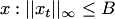, Vovk (2001) proves that for the square-loss function AAR can achieve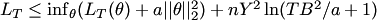,

where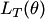is the loss of any linear function of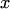.

The algorithm works as follows (all the vectors are columns):

Initialize vector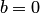, and matrix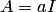;
FORRead new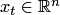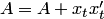Output prediction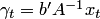Read new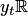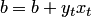END FOR.

This algorithm almost repeats online Ridge Regression (RR), with the difference that in RR the matrix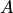is updated after the prediction has made. This means the method chooses to follow the best expert. Unfortunately, it there is no theoretical guarantees for RR in the same form as for AAR.

AAR adds an additional term to the RR minimization function: on the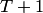step its predictor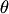achieves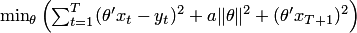, where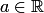.

### Bibliography

• Vladimir Vovk. Competitive on-line statistics. International Statistical Review 69, 213–248 (2001).